## Moment-Generating Function

Given a Random Variable, if there exists ansuch that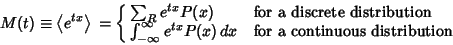(1)

for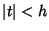, then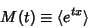(2)

is the moment-generating function.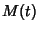(3)

where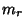is the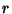th Moment about zero. The moment-generating function satisfies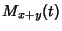(4)

Ifis differentiable at zero, then theth Moments about the Origin are given by(5)(6)(7)(8)

The Mean and Variance are therefore(9)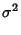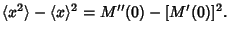(10)

It is also true that(11)

whereand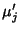is the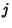th moment about the origin.

It is sometimes simpler to work with the Logarithm of the moment-generating function, which is also called the Cumulant-Generating Function, and is defined by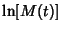(12)(13)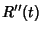(14)

But, so(15)(16)

Kenney, J. F. and Keeping, E. S. Moment-Generating and Characteristic Functions,'' Some Examples of Moment-Generating Functions,'' and Uniqueness Theorem for Characteristic Functions.'' §4.6-4.8 in Mathematics of Statistics, Pt. 2, 2nd ed. Princeton, NJ: Van Nostrand, pp. 72-77, 1951.